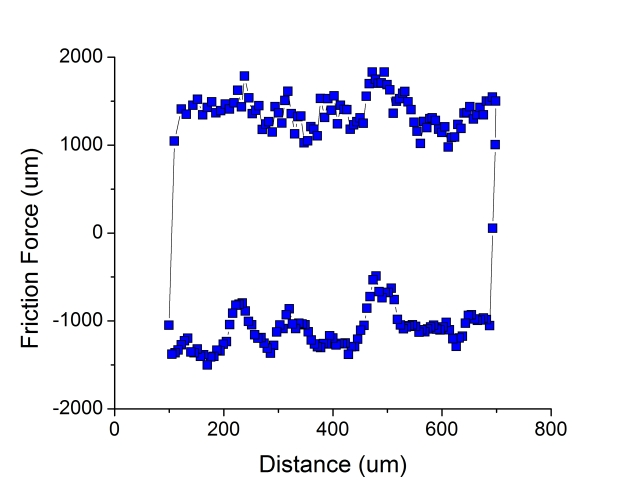## Introduction to Tribology

Friction

Friction is, by definition, the resistance to motion. The magnitude of this resistance is a function of the materials, geometries and surface features of the bodies in contact, as well as the operating conditions and environment. It is often desirable to minimize friction to order to maximize the efficiency of a component or process. Generally speaking, friction increases with load and surface roughness and can be decreased by the use of a lubricant.

Friction is the tangential resistance to motion between two contacting solid bodies. In 1699, Amontons proposed two "laws" of friction: (1) friction force is independent of nominal (or apparent) contact area between the two bodies, and (2) friction force is directly proportional to the surface-normal component of load. The second of these two laws gives us the equation F = μ W, where F is the friction force, W is the load and μ is the friction coefficient. To understand this relationship, consider the inclined plane experiment shown in the figure on the left below. As the plane is gradually tilted up and the incline angle Θ is increased, the component of the force due to the weight of the block in the direction of sliding increases. The friction coefficient, which is the ratio of the friction force F to the normal force W, is simply equal to tan Θ. Also, the magnitude of the friction before sliding begins is always greater than that during sliding. This difference is illustrated in the figure on the right below, where the pre-sliding friction is called the static friction and the friction after sliding begins is called the kinetic friction.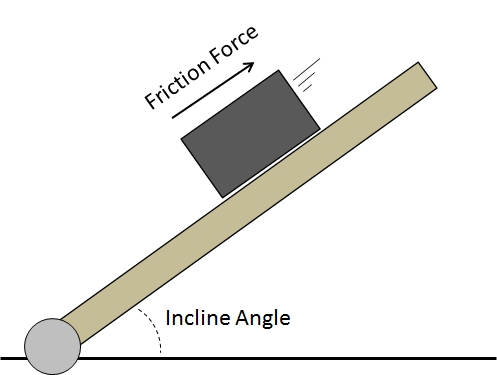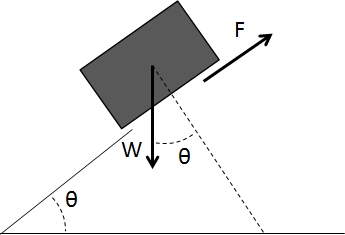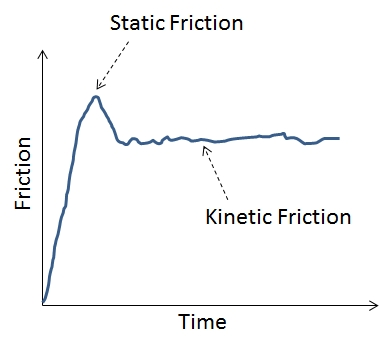In reality, friction is not just a function of load and angle, but is also a complex function of the material and surface properties of the two contacting bodies. Friction is measured using an instrument called a tribometer, where the lateral force (friction) and normal force (load) are measured while one body moves relative to another. Most tribometers measure friction either during reciprocating or unidirectional motion. As illustrated in the figure below, reciprocating motion occurs when one body is slid back and forth over another and unidirectional motion occurs when one body travels through a circular path on the other. The tribometer in our lab, shown below on the right, uses reciprocating motion to measure friction.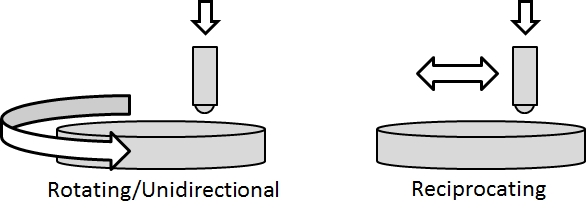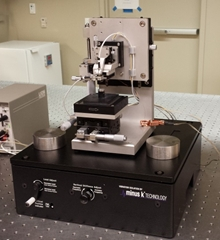An example of a friction loop is shown below, where the sign of the friction force reflects the direction in which the force acts, opposite to the direction of motion. This result is from a single cycle, where a cycle includes one forward stroke and one backward stroke. In this data, we see some evidence of stick-slip friction where the relative motion occurs through abrupt starting and stopping, called stick-slip, such that the friction loop exhibits repeated patterns of both static and kinetic friction. The friction coefficient for a given cycle is typically taken as the average of the forward and backward stroke data in the middle of the friction loop, i.e. away from the ends where inertial effects may play a role. An example of the average friction coefficient per cycle for three different samples is also shown below. We observe that the friction initially decreases with cycle for all the samples. This is typical and is called run-in, a process where the surfaces of the two bodies are evolving to accommodate sliding. After run-in, we have steady-state friction in which, assuming there is no significant wear, the friction does not change with additional sliding cycles. We observe different steady-state friction for these three samples, which is attributable to differences in their material and surface properties.